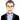# Building a Lightweight Speedometer in React Native

January 12, 2019

#### Contents

• Basic Requirements
• Default Props Overview
• Utils
• Integrating into the Component
• Usage

### Basic Requirements

The only external dependency required is the prop-types package for Type Checking or Prop Validation.

Other than that, we need one custom speedometer needle image.

### Default Props Overview

``````  defaultValue: 50,
minValue: 0,
maxValue: 100,
easeDuration: 500,
labels: [
{
name: 'Too Slow',
labelColor: '#ff2900',
activeBarColor: '#ff2900',
},
{
name: 'Very Slow',
labelColor: '#ff5400',
activeBarColor: '#ff5400',
},
{
name: 'Slow',
labelColor: '#f4ab44',
activeBarColor: '#f4ab44',
},
{
name: 'Normal',
labelColor: '#f2cf1f',
activeBarColor: '#f2cf1f',
},
{
name: 'Fast',
labelColor: '#14eb6e',
activeBarColor: '#14eb6e',
},
{
name: 'Unbelievably Fast',
labelColor: '#00ff6b',
activeBarColor: '#00ff6b',
},
],
needleImage: require('./images/speedometer-needle.png'),``````

value: Current value

defaultValue: Initial value

minValue: Minimum limit

maxValue: Maximum limit

easeDuration: Ease duration of the needle animation

labels: List of labels with color

needleImage: Absolute path to the needle image

### Utils

There are three types of Utility functions that are required.

• Calculate Degree from Labels
• Calculate Labels from Value
• Limit Value

#### Calculate Degree from Labels

This Simple Utility function will return the per label degree from the list of labels.

``````  function calculateDegreeFromLabels(degree, labels) {
const perLevelDegree = (degree) / (labels.length);
return perLevelDegree;
}

export default calculateDegreeFromLabels;``````

The total degree of the circle is divided by labels length to give per level degree.

#### Calculate Labels from Value

This Utility function will return the current label from the list of labels based on the current value of the speedometer.

``````  function calculateLabelFromValue(value, labels, minValue, maxValue) {
const currentValue = (value - minValue) / (maxValue - minValue);
const currentIndex = Math.round((labels.length - 1) * currentValue);
const label = labels[currentIndex];
return label;
}``````

First, the value of the speedometer is normalized to a percentage based on the `maxValue` and the `minValue`.

Then the current index of the label is calculated by multiplying it to the length of the labels list. (The index is always 1 less than the actual).

At last, we can return the label from the list based on the calculated index.

#### Limit Value

This Utility function is used to limit the current value between `maxValue` and `minValue` .

``````  function limitValue(value, minValue, maxValue, allowedDecimals) {
let currentValue = 0;
if (!isNaN(value)) {
if (!isNaN(allowedDecimals) && allowedDecimals > 0) {
currentValue = parseFloat(value).toFixed(allowedDecimals < 4 ? parseInt(allowedDecimals) : 4);
} else {
currentValue = parseInt(value);
}
}
return Math.min(Math.max(currentValue, minValue), maxValue);
}``````

### Integrating into the Component

``````  wrapper: {
marginVertical: 5,
alignSelf: 'center',
},
// Circular Container
circleWrapper: {
overflow: 'hidden',
},
outerCircle: {
justifyContent: 'flex-end',
alignItems: 'center',
overflow: 'hidden',
borderColor: '#ffffff',
backgroundColor: '#e6e6e6',
},
halfCircle: {
position: 'absolute',
top: 0,
left: 0,
},
imageWrapper: {
position: 'absolute',
left: 0,
zIndex: 10,
},
image: {
resizeMode: 'stretch',
height: width - 20,
width: width - 20,
},
innerCircle: {
overflow: 'hidden',
justifyContent: 'flex-end',
alignItems: 'center',
backgroundColor: '#ffffff',
width: width * 0.6,
height: (width / 2) * 0.6,
borderTopLeftRadius: width / 2 - 10,
borderTopRightRadius: width / 2 - 10,
},
labelWrapper: {
marginVertical: 5,
alignItems: 'center',
},
label: {
fontSize: 25,
fontWeight: 'bold',
},
labelNote: {
fontSize: 16,
fontWeight: 'bold',
},``````

wrapper: Wrapper styles.

outerCircle: Outer Circle styles.

innerCircle: Inner Circle styles.

halfCircle: Used to build label blocks between outer and inner circle.

imageWrapper: Speedometer Needle Image Wrapper styles.

image: Speedometer Needle Image styles.

label: Label styles.

labelNote: Label note styles.

#### Constructing the static component based on the styles

``````  {labels.map((level, index) => {
const circleDegree = 90 + (index * perLevelDegree);
return (
<View
key={level.name}
style={[style.halfCircle, {
backgroundColor: level.activeBarColor,
width: currentSize / 2,
height: currentSize,
transform: [
{ translateX: currentSize / 4 },
{ rotate: `\${circleDegree}deg` },
{ translateX: (currentSize / 4 * -1) },
],
}, halfCircleStyle]}
/>
);
})}``````

By adding this your, component speedometer will look like this

The reason to add style objects in an array is that we’ll be appending more styles to it later on.

``````  const degree = 180;
const perLevelDegree = calculateDegreeFromLabels(degree, labels);
const label = calculateLabelFromValue(
limitValue(value, minValue, maxValue, allowedDecimals), labels, minValue, maxValue,
);``````

The initial degree is set to 180 for a semi-circle.

Now we get the `perLevelDegree` and `label` based on the `value` supplied as the props.

`circleDegree` is calculated for each `halfCircle` style based on the current label index and the `perLevelDegree`. `90` is added to it because our circle starts as a semi-circle.

The background color of our half circle is set based on the label that we get from our util.

Next we use transforms to position and rotate our block based on the `circleDegree`.

Adding the above changes will make the speedometer will something look like this

``````  Animated.timing(
this.speedometerValue,
{
toValue: limitValue(value, minValue, maxValue, allowedDecimals),
duration: easeDuration,
easing: Easing.linear,
useNativeDriver,
},
).start();

const rotate = this.speedometerValue.interpolate({
inputRange: [minValue, maxValue],
outputRange: ['-90deg', '90deg'],
});``````

First we need to initialize the speedometer value in the constructor. Then, we add the animations based on the current value limited by our utility function.

The output range is set in between `-90deg` to `90deg` for the needle to animate.

Now this component is ready to be exported and used.

### Usage

``````  state = {
value: 0,
};

onChange = value => this.setState({ value: parseInt(value) });

render() {
return (
<SafeAreaView style={styles.container}>
<TextInput placeholder="Speedometer Value" style={styles.textInput} onChangeText={this.onChange} />
<RNSpeedometer value={this.state.value} size={200} />
</SafeAreaView>
);
}``````

After making the necessary changes and updating value based on the `TextInput` the component will look like this.The complete code can be found on

as react-native-speedometer package.